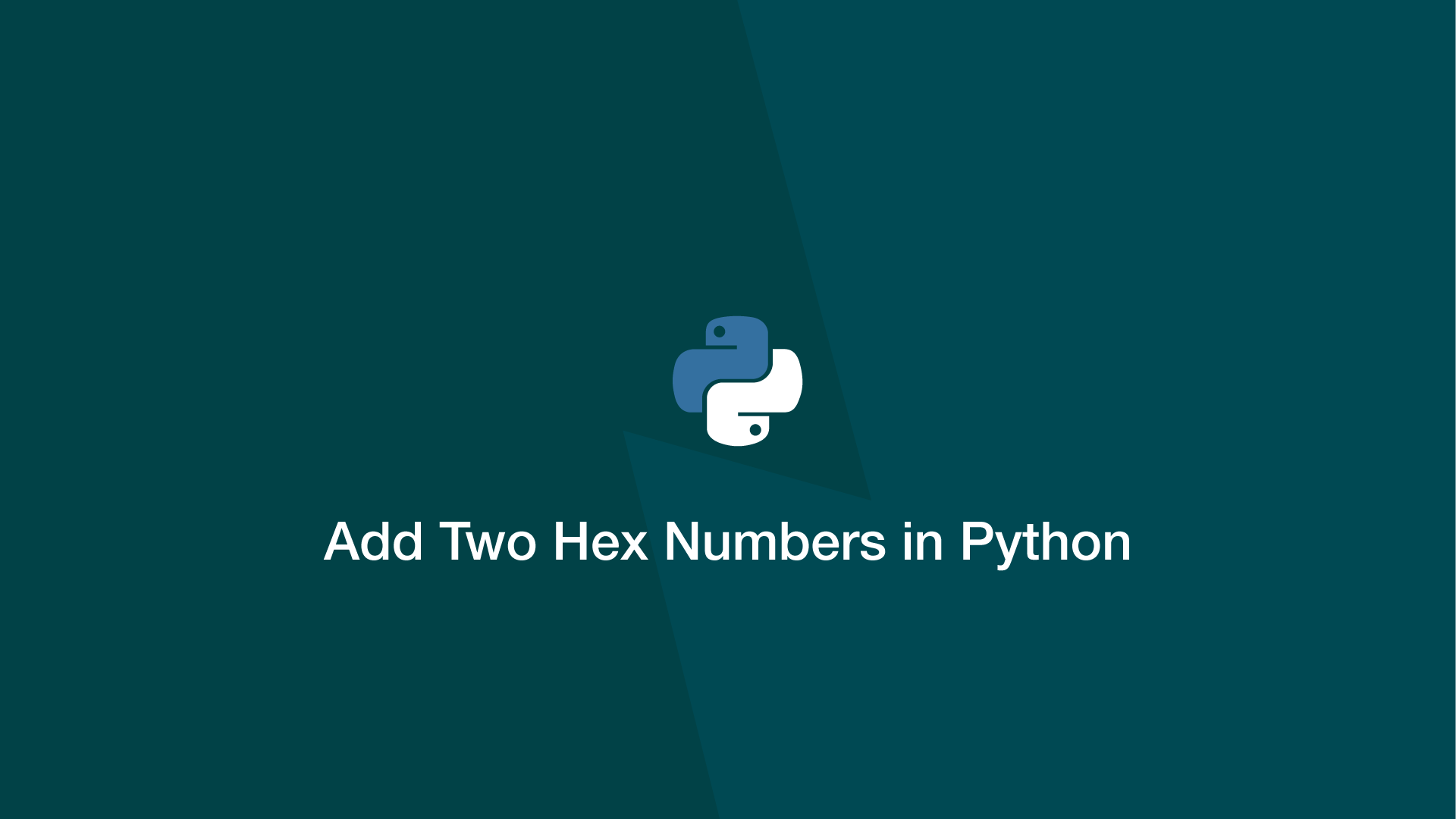# How to Add Two Hex Numbers in PythonTo add hexadecimal numbers in Python, first, convert them into decimals, perform the operation then convert the result back into the base 16 numeric system.

Let's look at an example of converting two base 16 numbers to base 10 with the Python int() function, adding the values together, then converting the result to hexadecimal using the Python hex() function.

``````a = '8AB'
b = 'B78'

a_int = int(a, 16)
b_int = int(b, 16)

result = hex(a_int + b_int)

print(result[2:])
``````
``````1423
``````

The whole operation could be done on one line and remain readable:

``````a = '8AB'
b = 'B78'

result = hex(int(a, 16) + int(b, 16))

print(result[2:])
``````
``````1423
``````

If you need to add hex numbers more than once in a program it might be worth creating a function to reduce code repetition. Here is an example of how that might look:

``````def add_hex(a, b):
return hex(int(a, 16) + int(b, 16))

``````
``````1423
``````

#### Related Tutorials### How to Use Argparse in Python

September 25, 2020### How to Convert a String from Hex to ASCII in Python

April 27, 2021### Convert Hexadecimal to Decimal in Python

November 22, 2021### How to use Sets in Python

September 15, 2020### How to Create and Use Lists (Arrays) in Python

September 01, 2020### How to Convert Hexadecimal to Binary in Python

November 22, 2021